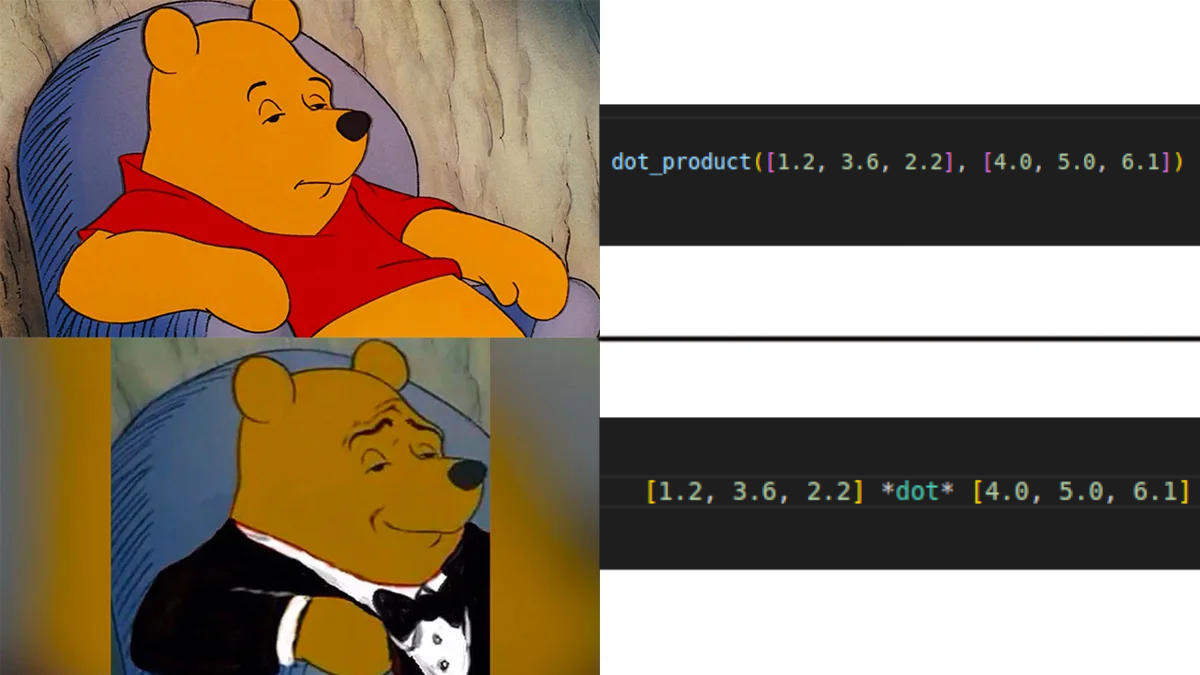Categories: Tech

Tags: RustI am not endorsing the code in this post.

## C++ Input/Output

``````stdin().read_line(&mut buffer).unwrap();
println!("Hello I am {name}!!!");
``````

we can overload the shift operators on `cin` and `cout` to allow

``````cin >> &mut buffer;
cout << "Hello I am " << name << "!!!" << endl;
``````

``````std::cmp::max(x, y);
[w, x, y, z].into_iter().max();
``````

we can make

``````// max+ is like std::cmp::max but better
// it supports >2 arguments
max+(x, y);
max+(w, x, y, z);
``````

## More Concise Builders

Here's a more serious one. Builder pattern sometimes involve a lot of repeated method calls. Take for example this usage of the warp web framework.

``````let hi = warp::path("hello")
.and(warp::path::param())
.map(|param: String, agent: String| {
format!("Hello {}, whose agent is {}", param, agent)
});
``````

What if the API look like this instead?

``````let hi = warp::path("hello")
+	warp::path::param()
>>	|param: String, agent: String| {
format!("Hello {}, whose agent is {}", param, agent)
};
``````

## Infix Functions

``````x.pow(y);
dot_product(a, b);
a.cross(b.cross(c).cross(d))
``````

we can make

``````x ^pow^ y;
a *dot* b;
a *cross* (b *cross* c *cross* d);
``````

Lots of people wanted this!

## Doublefish

`std::mem` provides these functions

``````size_of::<T>();
size_of_val(&value);
``````

Turbofish enthusiasts would enjoy `size_of` but not so much `size_of_val`, so let's make our own improved version of `size_of_val` that's more turbofishy

``````size_of::<T>();
size_of_val<<&value>>();
``````

## Join and Race

Futures combinators can have short-circuiting behaviors

``````// quit if any of the 3 errors
(fut1, fut2, fut3).try_join().await;

// quit if any of the 3 succeeds
(fut4, fut5, fut6).race_ok().await;
``````

let's communicate this through `&` and `|`

``````(TryJoin >> fut1 & fut2 & fut3).await;
(RaceOk >> fut4 | fut5 | fut6).await;
``````